Chapter 2 Class 10 Polynomials

Class 10
Important Questions for Exam - Class 10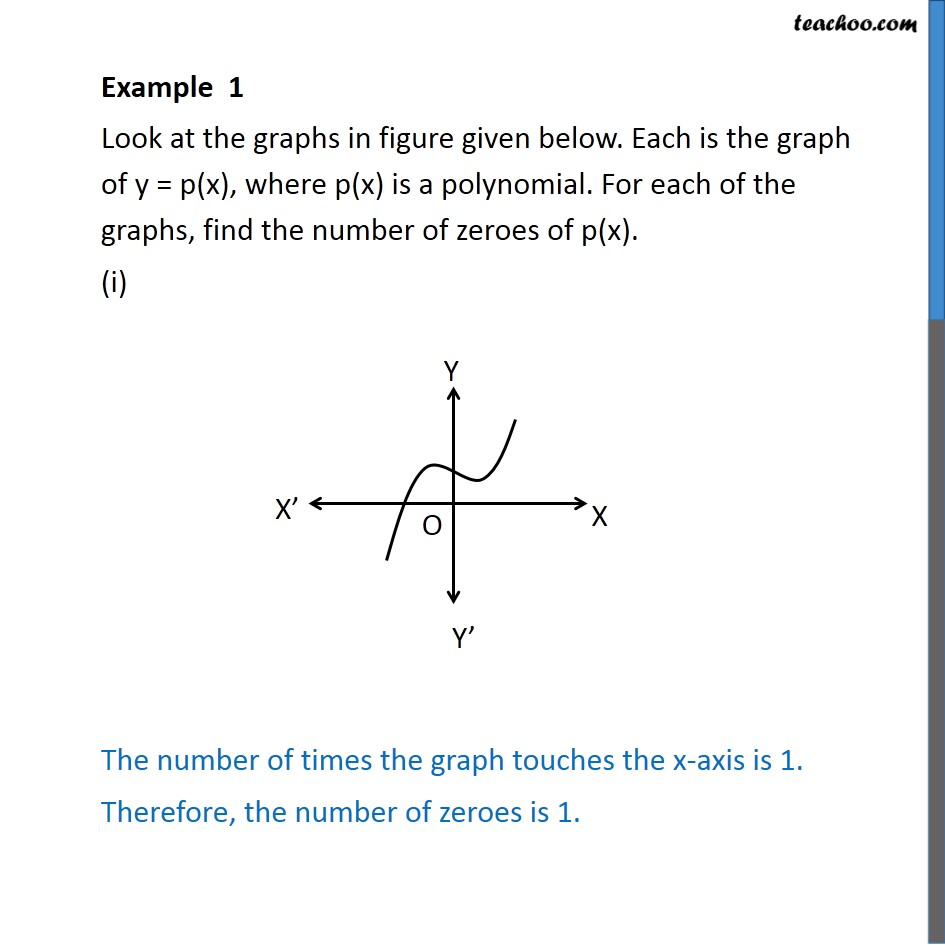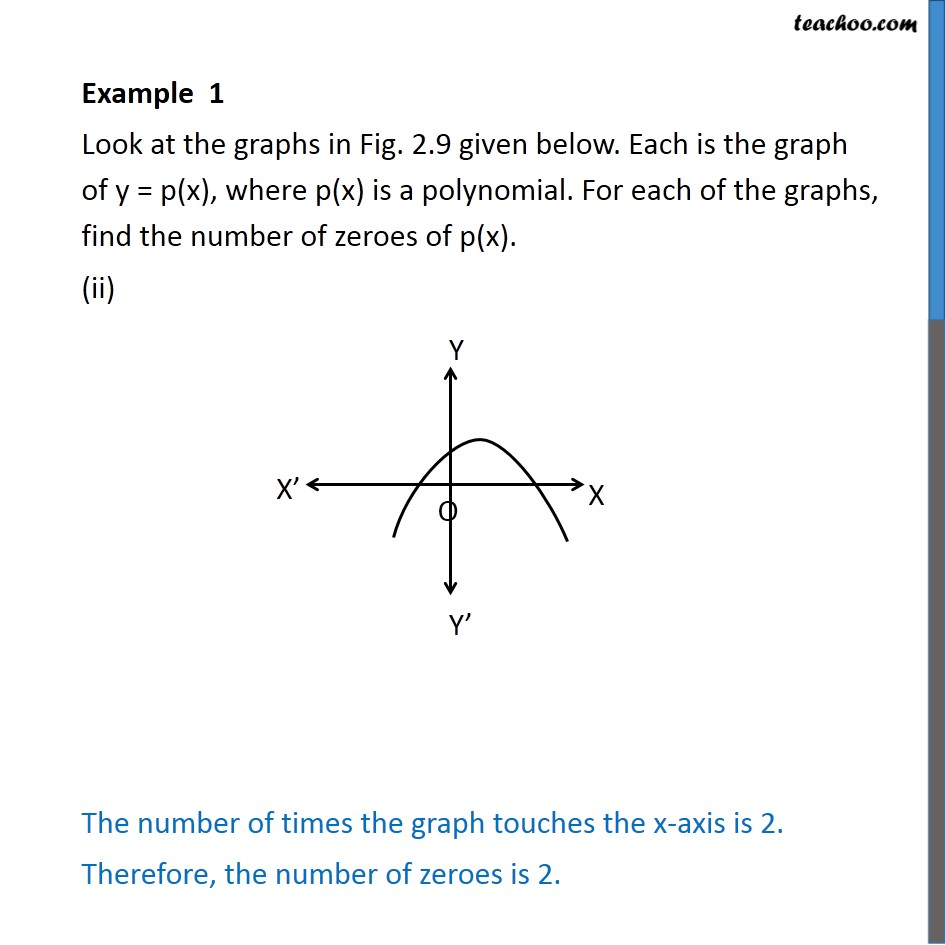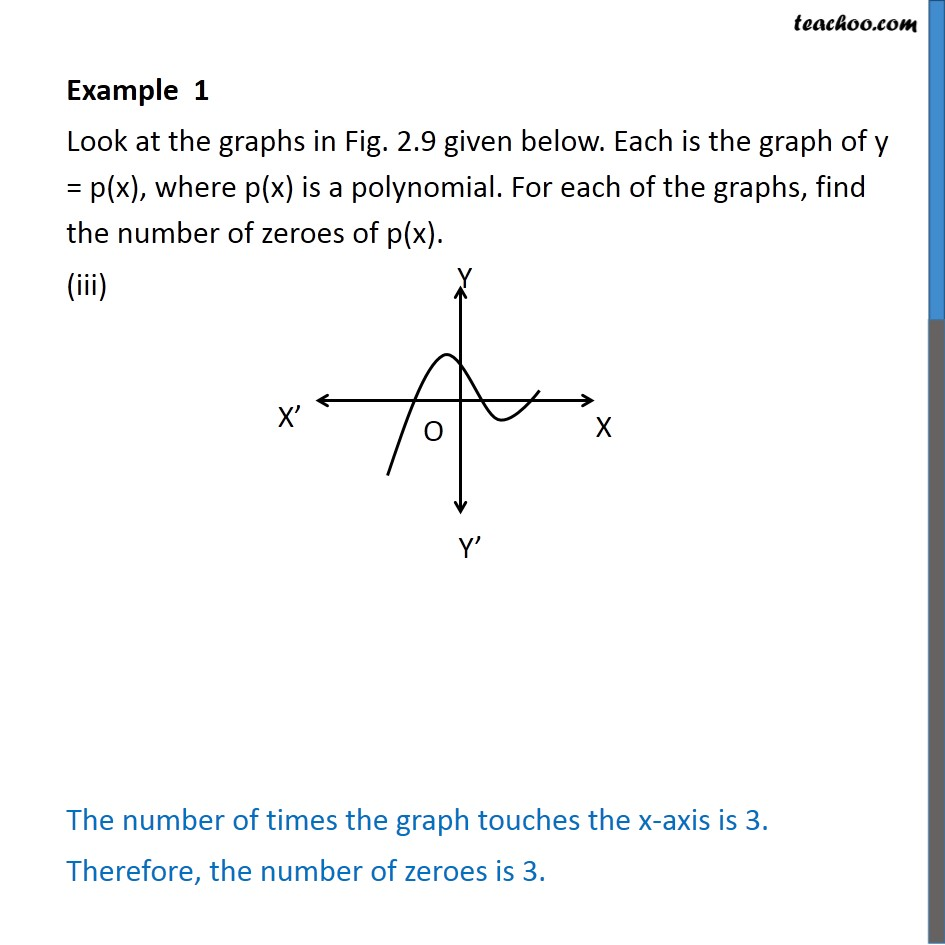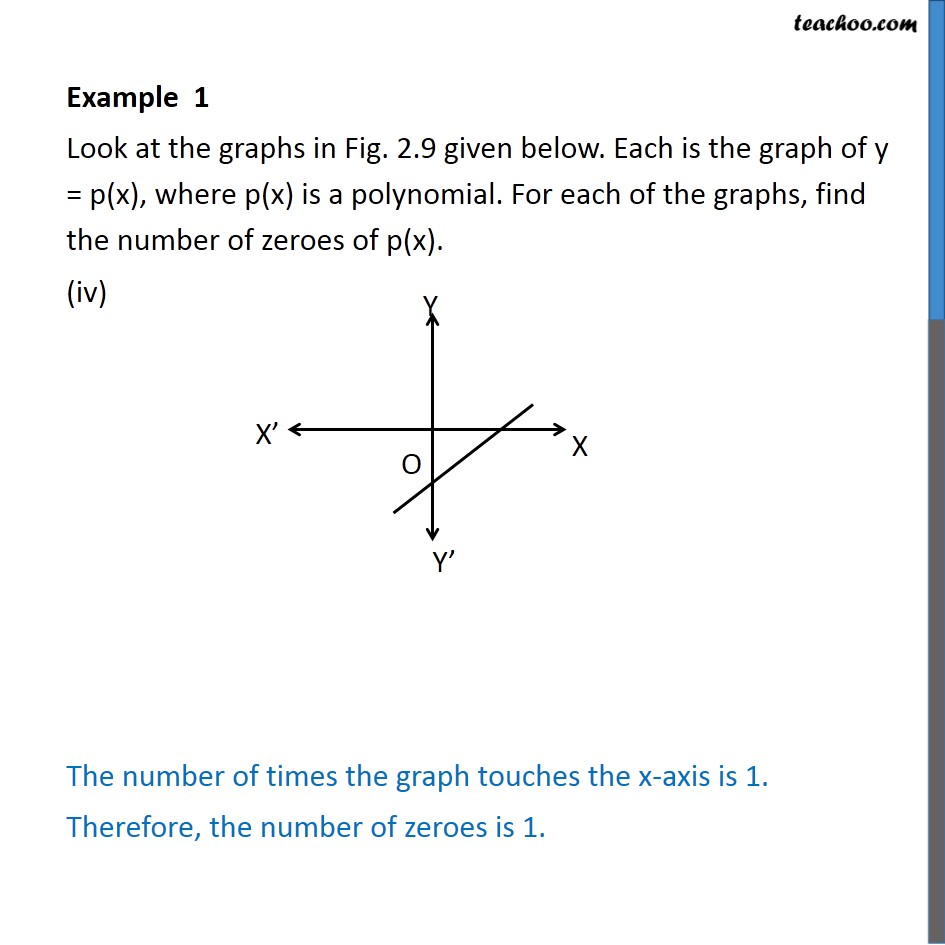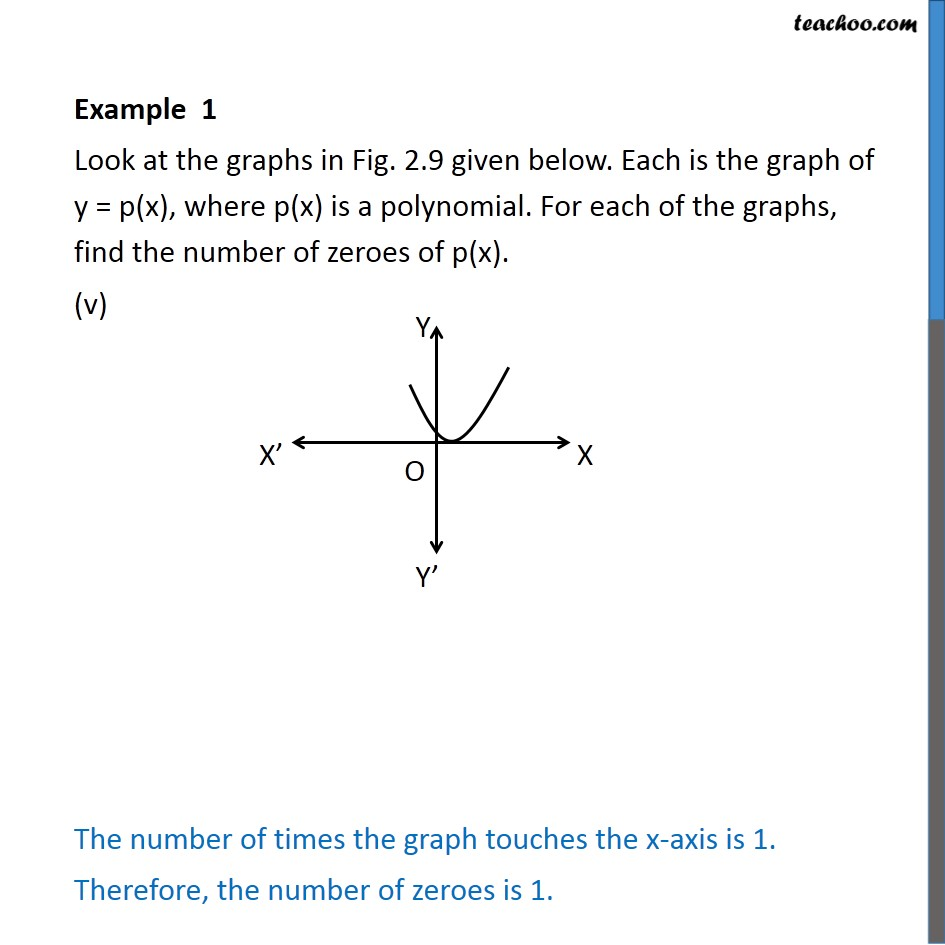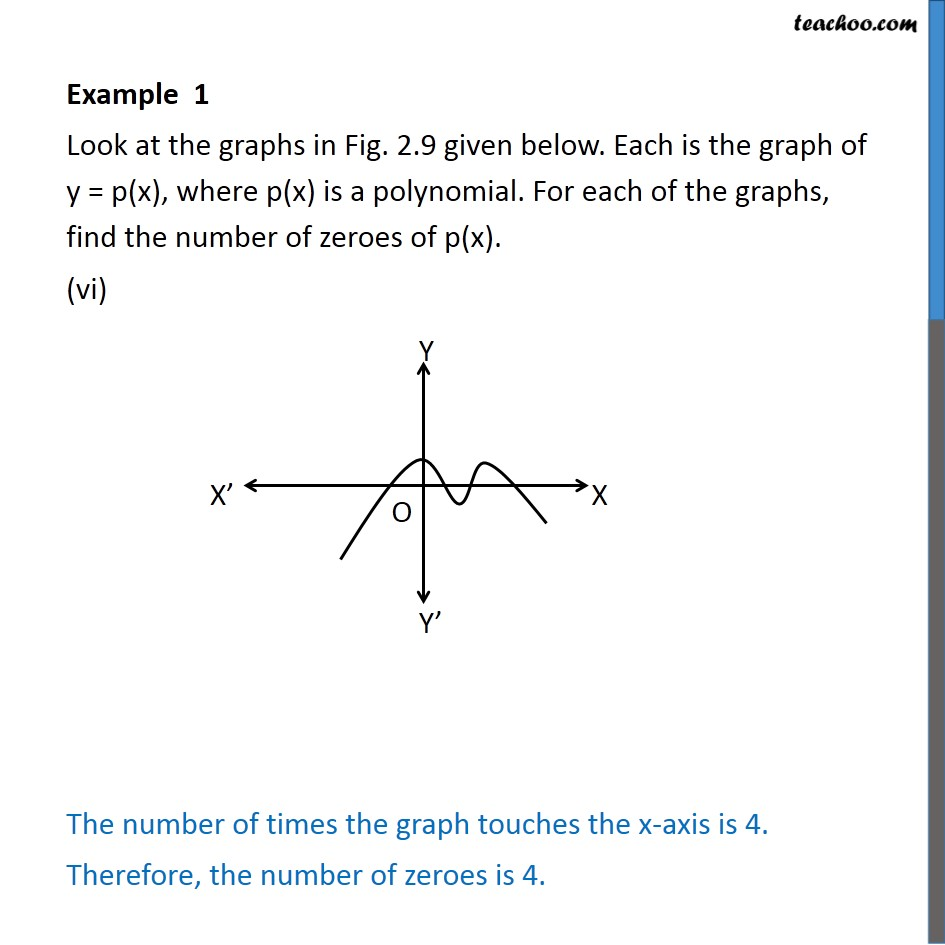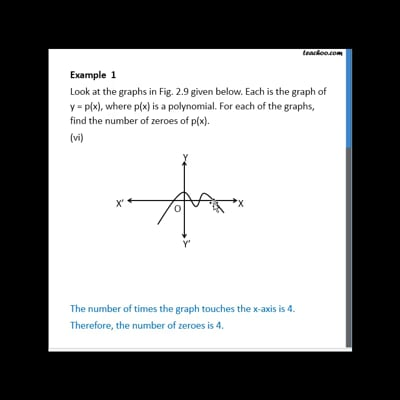This video is only available for Teachoo black users

### Transcript

Example 1 Look at the graphs in figure given below. Each is the graph of y = p(x), where p(x) is a polynomial. For each of the graphs, find the number of zeroes of p(x). (i) The number of times the graph touches the x-axis is 1. Therefore, the number of zeroes is 1. Example 1 Look at the graphs in Fig. 2.9 given below. Each is the graph of y = p(x), where p(x) is a polynomial. For each of the graphs, find the number of zeroes of p(x). (ii) The number of times the graph touches the x-axis is 2. Therefore, the number of zeroes is 2. Example 1 Look at the graphs in Fig. 2.9 given below. Each is the graph of y = p(x), where p(x) is a polynomial. For each of the graphs, find the number of zeroes of p(x). (iii) The number of times the graph touches the x-axis is 3. Therefore, the number of zeroes is 3. Example 1 Look at the graphs in Fig. 2.9 given below. Each is the graph of y = p(x), where p(x) is a polynomial. For each of the graphs, find the number of zeroes of p(x). (iv) The number of times the graph touches the x-axis is 1. Therefore, the number of zeroes is 1. Example 1 Look at the graphs in Fig. 2.9 given below. Each is the graph of y = p(x), where p(x) is a polynomial. For each of the graphs, find the number of zeroes of p(x). (v) The number of times the graph touches the x-axis is 1. Therefore, the number of zeroes is 1. Example 1 Look at the graphs in Fig. 2.9 given below. Each is the graph of y = p(x), where p(x) is a polynomial. For each of the graphs, find the number of zeroes of p(x). (vi) The number of times the graph touches the x-axis is 4. Therefore, the number of zeroes is 4.